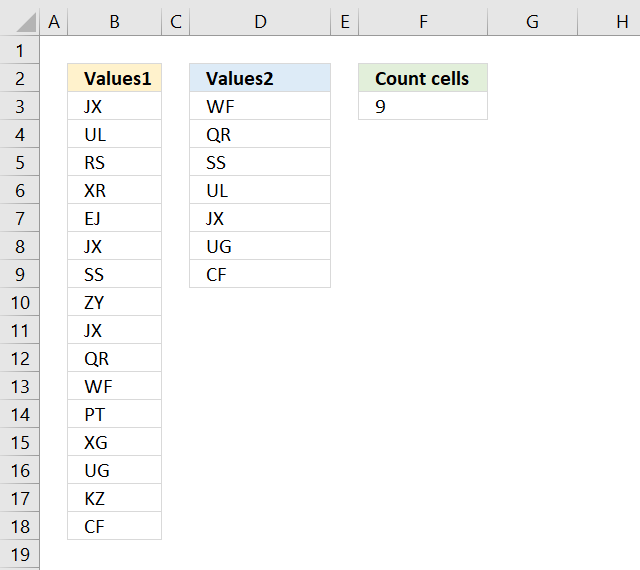Author: Oscar Cronquist Article last updated on October 02, 2018The formula in cell F9 counts the number of cells in column B (Values1) that are equal to any of the values in column D (Values2).

Formula in cell F9:

=SUMPRODUCT(COUNTIF(B3:B18,D3:D9))

### Explaining formula in cell F9

The COUNTIF function allows you to count cells in a range that are equal to a criterion. The great thing about the COUNTIF function is that it is possible to use criteria.

COUNTIF(B3:B18,D3:D9)

becomes

COUNTIF({"JX"; "UL"; "RS"; "XR"; "EJ"; "JX"; "SS"; "ZY"; "JX"; "QR"; "WF"; "PT"; "XG"; "UG"; "KZ"; "CF"}, {"WF"; "QR"; "SS"; "UL"; "JX"; "UG"; "CF"})

and returns the following array: {1; 1; 1; 1; 3; 1; 1}

The SUMPRODUCT function lets you sum the values in the array without the need to enter the fomula as an array formula.

SUMPRODUCT(COUNTIF(B3:B18,D3:D9))

becomes

SUMPRODUCT({1; 1; 1; 1; 3; 1; 1})

and returns 9 in cell F9. 1+1+1+1+3+1+1 = 9